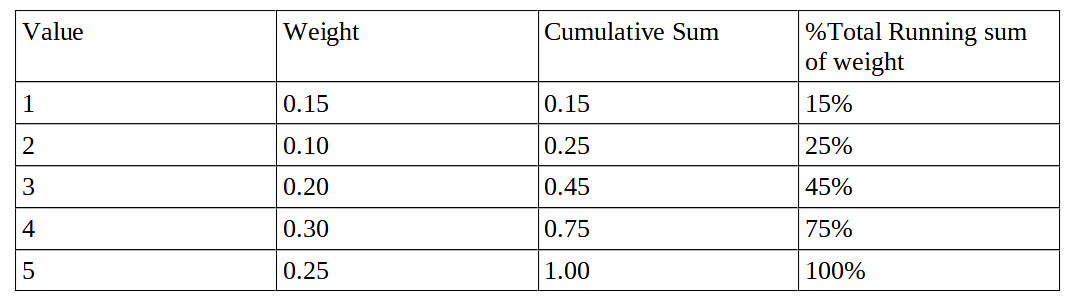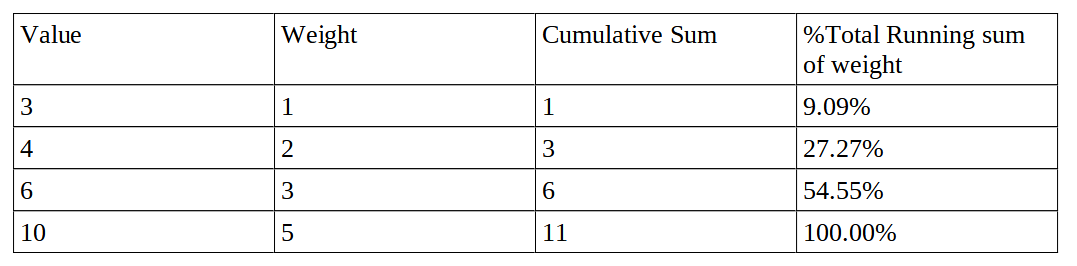×

Search anything:

# Weighted Median Problem

#### Algorithms Sorting AlgorithmsGet this book -> Problems on Array: For Interviews and Competitive Programming

In this article, we have presented the approach to solve the Weighted Median Problem efficiently and provided an implementation supporting the algorithm.

1. What is Weighted Median Problem?
2. Examples
3. Properties of Weighted Median
4. Concept of Lower weighted median and Upper Weighted Median
5. Algorithm
6. Implementation in C++
7. Code Explanation
8. Time and Space Complexity

## What is Weighted Median Problem?

Weighted Median is a measure of central tendency which is better than normal median. In this let us consider a weighted median value ‘wi’ when the elements are sorted such that the total weight of the elements to the left of wi is half of the total weight. Or else such element will be chosen as pivot whose total weights on left and right side will be of the least difference possible after crossing the cumulative sum value of 50% of total.

## Examples

Example:1It can be seen from the table that the weighted median element is 4 because at the weight corresponding to element 4, the total cumulative sum passes 50%.

Example:2Let us consider a set of numbers 3,4,6,10 with weights 1,2,3,5 then the median would be 6, since (1+2+3)/(1+2+3+5) = 6/11 = 54.55%.

## Properties of Weighted Median

1. The cumulative sum of the weights on each side of the pivot should be as equal as possible.
2. If weights of all elements are equal then weighted median and normal median is the same entity.

## Concept of Lower weighted median and Upper Weighted Median

This concept is applied only when there are even number of elements.
Lower Weighted Median: When the cumulative sum from Lower(1,2,3....) side of the partition up to that element is exactly half of the total sum of weights then it is called the lower weighted median.

Upper Weighted Median: When the cumulative sum from higher(5,4,3...) side of partition up to the element is exactly half of the total sum of weights then it is called the upper weighted median.

## Algorithm

1. Sort the elements with a optimised approach.
2. Scan the elements from the lowest to the highest element while calculating the cumulative sum of the weights till the current index.
If (n is odd)
3. if sum>=1/2*total then the current index is the index of the weighted median element.
Else go to the next element.
Else{
4. if (condition of lower and upper weighted median arises then) {
Iterate from lowest to highest to find lower weighted median and highest to lowest to find upper
weighted median.
}
else
{
repeat the same as step 3
}
5. Return the element.

## Implementation in C++

``````#include<bits/stdc++.h>
using namespace std;

struct Node{

double ele;
double wt;

};

bool compare(Node p, Node q){
return p.ele<q.ele;
}

int main(){
int n;
cout<<"Enter no of elements: "<<endl;
cin>>n;
Node med[n];
cout<<"Enter element and weight: "<<endl;
for(int i=0; i<n; i++){
cin>>med[i].ele;
cin>>med[i].wt;
}
cout<<"Sorted list is:  "<<endl;
sort(med, med+n, compare);
for(int i=0; i<n; i++){
cout<<med[i].ele<<" "<<med[i].wt<<endl;
}
double sum=0;
for(int i=0; i<n; i++){
sum= sum + med[i].wt;
}
double sum2=sum/2, sum3=0;
if(n%2!=0){
for(int i=0; i<n; i++){
sum3=sum3+med[i].wt;
if(sum3>=sum2){
cout<<"Weighted median :"<<med[i].ele<<endl;
return 0;
}

}

}
else{
for(int i=0; i<n; i++){
sum3=sum3+med[i].wt;
if(sum3>=sum2){
cout<<"Lower Weighted median :"<<med[i].ele<<endl;
break;
}
}
sum3=0;
for(int i=n-1; i>=0; i--){
sum3= sum3 + med[i].wt;
if(sum3>=sum2){
cout<<"Upper weighted median :"<<med[i].ele<<endl;
return 0;
}
}
}

return 0;
}
``````

Output: Example 1

``````Enter no of elements:
4
Enter element and weight:
1 0.25
2 0.25
3 0.25
4 0.25
Sorted list is:
1 0.25
2 0.25
3 0.25
4 0.25
Lower Weighted median : 2
Upper weighted median : 3
``````

Output: Example 2

``````Enter no of elements:
5
Enter element and weight:
3 0.2
4 0.3
1 0.15
2 0.1
5 0.25
Sorted list is:
1 0.15
2 0.1
3 0.2
4 0.3
5 0.25
Weighted median element : 4
``````

## Code Explanation

1. A struct was made for storing element value and weight.
2. Input the number of elements along with the elements and their respective weight.
3. Apply the sort function to sort the array of the struct according to the element values.
4. Calculate the total sum of the weights.
5. check if there are even or odd number of elements
6. If odd then check for the element where the cumulative sum crosses totalsum/2
7. Else traverse from both the sides to find lower weighted median and upper weighted median
and if both are different then it means there are two pivot elements from where the cumulative sum is exactly half of the totalsum.
8. Return the weighted median element.

## Time and Space Complexity

Space Complexity is O(n) since the size of array depends on the number of elements.
Time Complexity mainly depends on the way the sorting is done as all other loops require O(n) of time so if the sorting is done with time complexity of O(nlogn) then the time complexity of the program would be O(n*logn) as well.

With this article at OpenGenus, you must have the complete idea of Weighted Median Problem and how to solve it.